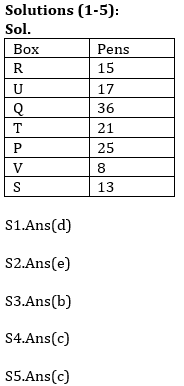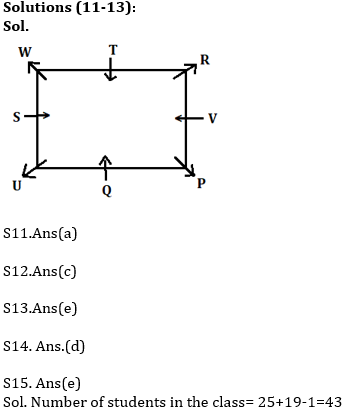Latest Banking jobs   »   NIACL AO 2021 Result Out, Mains Exam Date For 300 AO Recruitment   »   Reasoning Ability Quiz For IBPS Clerk/NIACL...

# Reasoning Ability Quiz For IBPS Clerk/NIACL AO Prelims 2021- 18th September

Directions (1-5): Study the information carefully and answer the questions given below.
Seven boxes P, Q, R, S, T, U and V are placed one above other in a stack. Each box contains different numbers of pens i.e. 21, 17, 13, 25, 36, 8 and 15. All information is not necessarily in same order.
Two boxes are placed between P and U. The number of pens in box U is a prime number. Box R placed just above the box which contain 17 pens. Box V is placed just below box P and contains lowest number of pens. Two boxes are placed between box V and box Q, which contains 36 pens. Box P contains 8 pens more than box U. Box P does not contain less than 22 pens. Two boxes are placed between box S and box T. Box T contain more numbers of pen than box S. Box Q does not placed just above and just below box S. The number of pens in box Q is perfect square of a number. Box R contains number of pens that is multiple of 5.

Q1. Which of the following box is placed just above the box which contains 36 pens?
(a) R
(b) P
(c) T
(d) U
(e) None of these

Q2. Which of the following box contains 13 pens?
(a) T
(b) Q
(c) V
(d) R
(e) None of these

Q3. Which of the following pair of boxes is placed just above and just below respectively of box T?
(a) U, P
(b) Q, P
(c) R, Q
(d) P, S
(e) None of these

Q4. Four of the following five are alike in certain way based from a group, find the one that does not belong to that group?
(a) U-21
(b) Q-25
(c) R-13
(d) T-8
(e) S-25

Q5. How many boxes are placed between box U and Box S?
(a) Two
(b) Three
(c) Four
(d) More than Four
(e) None

Directions (6-10): Study the following alphanumeric series carefully and answer the questions given below:

9 U 1 Q * 7 B \$ * G & A N # E 3 V @ 6 R 6 X 3 2 D 8 % W 2 4

Q6. How many such numbers are there which is preceded by symbols and followed by alphabets?
(a) Two
(b) Four
(c) Three
(d) One
(e) None

Q7. Which of the following element is 21th from right end of the series?
(a) &
(b) *
(c) G
(d) N
(e) None of these

Q8. How many such symbols are there which is preceded by numbers and followed by vowels?
(a) Two
(b) None
(c) One
(d) Four
(e) Three

Q9.How many such alphabets are there which is preceded and followed by same number?
(a) Three
(b) Two
(c) One
(d) Four
(e) None

Q10. If all numbers are eliminated from the series then, which of the following element is 9th from right end of the series?
(a) N
(b) A
(c) E
(d) #
(e) None of these

Directions (11-13): Study the information carefully and answer the questions given below.
Eight persons i.e. P, Q, R, S, T, U, V and W are sitting in a square table in such a way that the Four persons sit at four corner and face outside and four persons who sit at middle of the side of square table face inside. S sits third to the right of P. T sits second to left of S. W sits second to left of R. Only one person sits between Q and V. W face outside. The person who faces Q sits third to the right of U.

Q11. Who among the following sits immediate left of U?
(a) Q
(b) S
(c) T
(d) V
(e) None of these

Q12. How many persons sit between W and Q, when counted to the right of Q?
(a) Two
(b) Three
(c) More than Three
(d) One
(e) None

Q13. Who among the following sits third to the left of R?
(a) Q
(b) T
(c) W
(d) P
(e) None of these

Q14. In a certain code, FINANCIAL is written as ZMRUMOZRX. How is INTERVIEW written in the code?
(a) VGMRGDVRE
(b) RIVGMDVRE
(c) VGIDVREMR
(d) VGMRIDVRE
(e) None of these

Q15. Rahul ranked 25th from the top and 19th from the bottom in a class. How many students are there in the class?
(a) 44
(b) 40
(c) 33
(d) 35
(e) None of these

SolutionsS6. Ans(a)
Sol. (*7B) (@6R)

S7. Ans(c)

S8. Ans(b)

S9. Ans(c)

S10.Ans(d)Click Here to Register for Bank Exams 2021 Preparation Material#### Congratulations!Download Hindu Review of October 2021: Free PDF